Published

# MEGR3171 Tamper-Sensing Secure Box

An IOT-connected locking box for valuables that sends an alert and remotely sets off an alarm if moved.

IntermediateShowcase (no instructions)358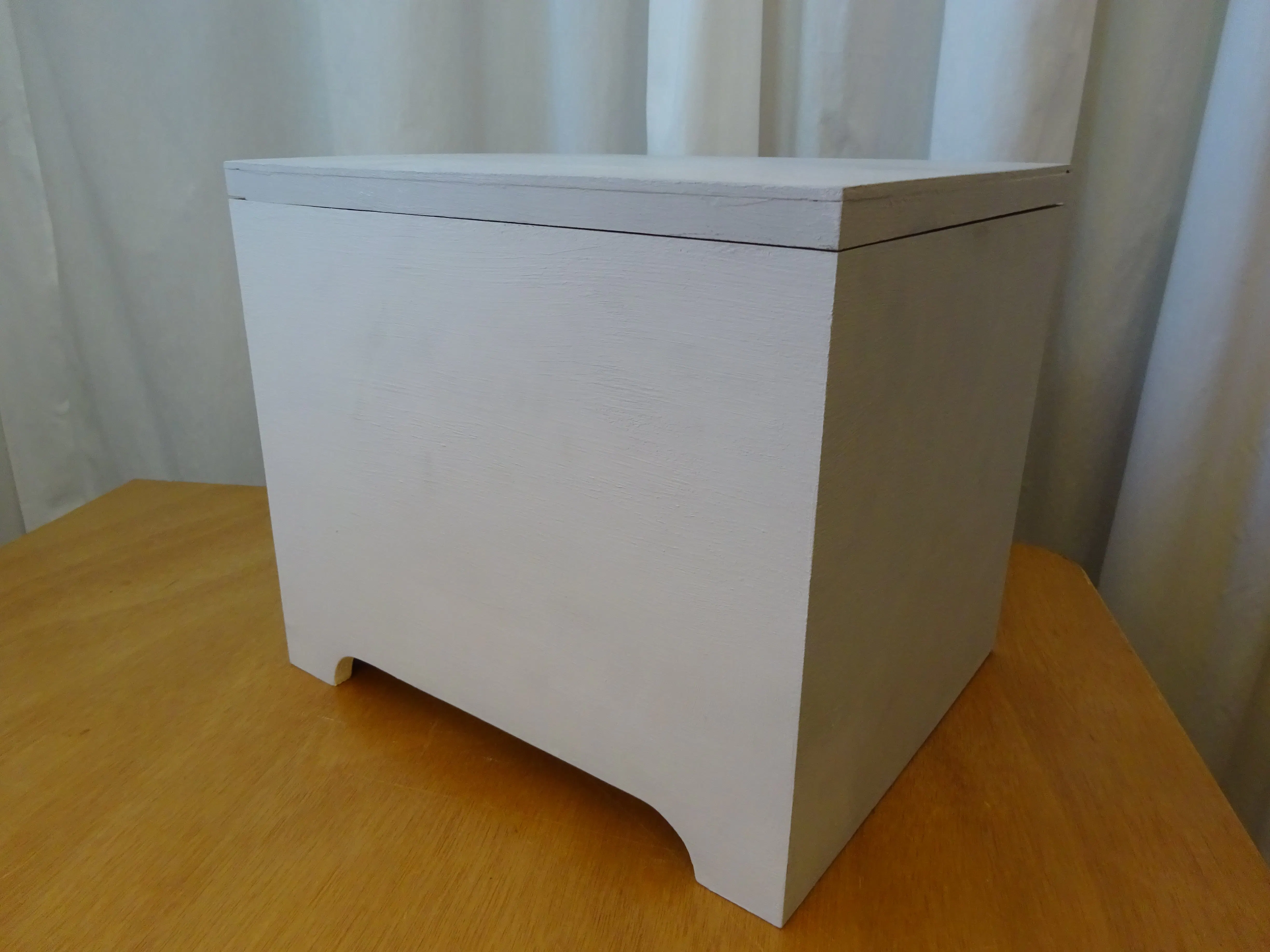## Things used in this project

### Hardware components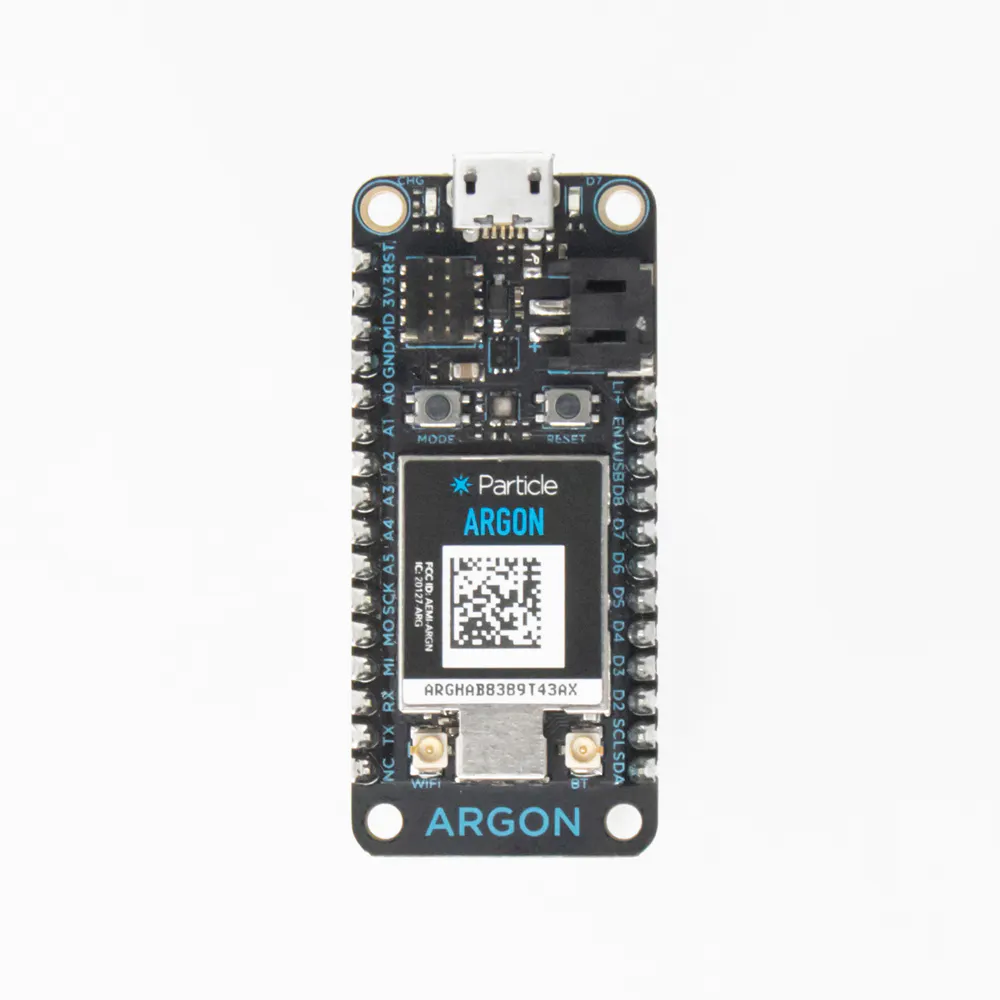Particle Argon
×2Ultrasonic Sensor - HC-SR04 (Generic)
×1
 Uxcell DC5V Push Type Electromagnetic Solenoid Lock
×1Buzzer Part CEM-1203(42)
×2
 Anker Astro E1 5200mAh External Battery
×1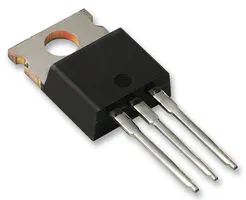Darlington High Power Transistor
×1
 1N4001 Diode
×1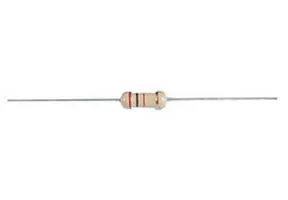Resistor 220 ohm
×1

### Software apps and online servicesParticle Build Web IDEIFTTT Maker service## Custom parts and enclosures

### Secure box assembly

Example model of box in Fusion 360

### Solenoid bracket

Bracket to attach the solenoid to the box lid or any other flat surface

## Schematics

### Box circuit

The circuit that is inside the secure box, which controls the look and position sensor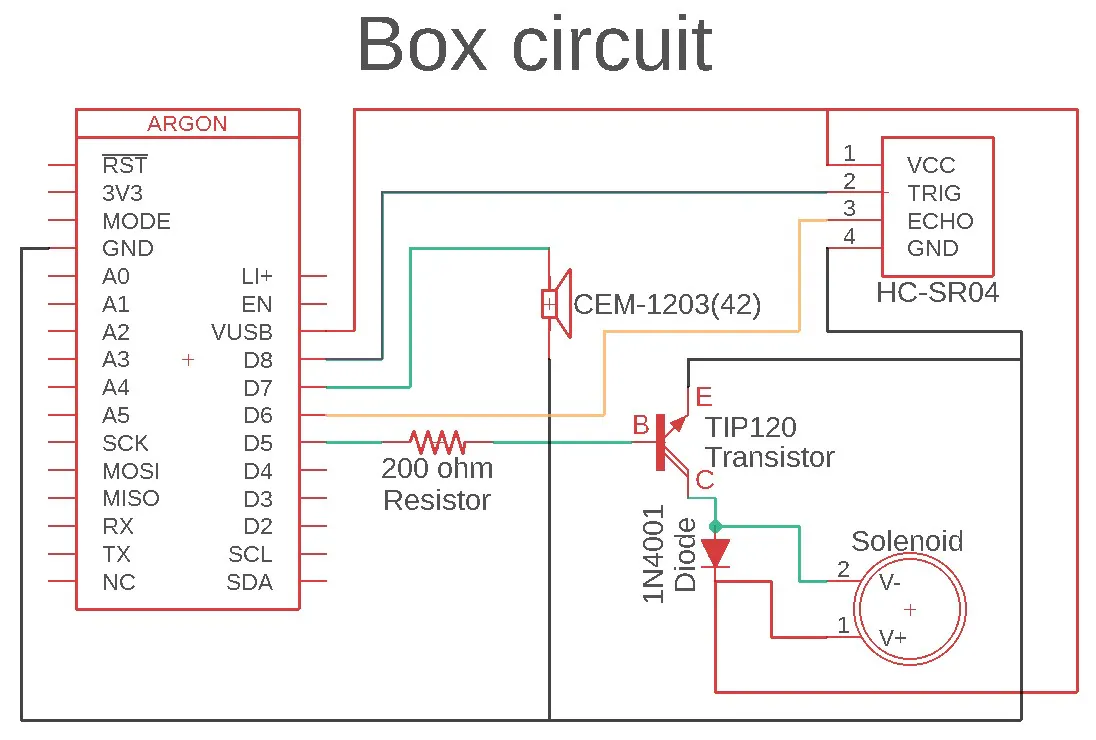### Transceiver circuit

The circuit that looks for a published event from the box.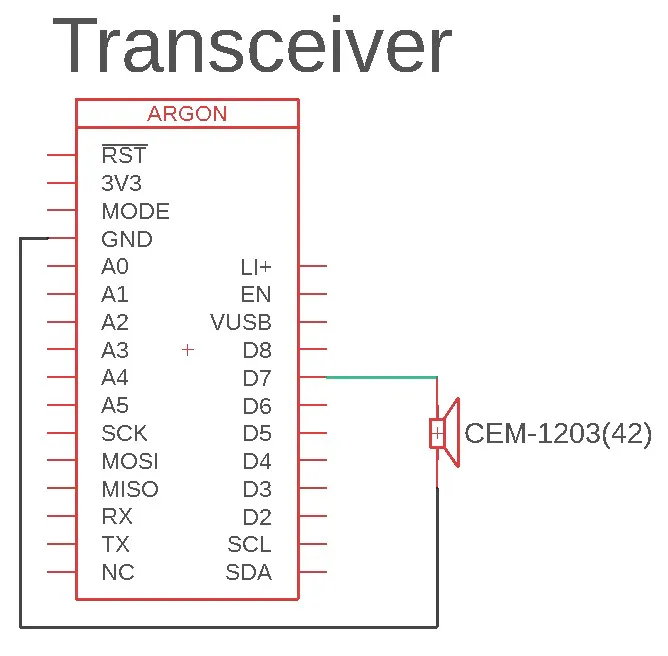## Code

### Transceiver code

Arduino
This is the code for the transceiver argon
```int buzzer = 7; //allows for labeling pin
int n=0; int o=0; int p=0; int f=0; int s=0; int i = 0; int a=0;//sets up variables

void setup(){
Particle.subscribe("box moved", alarmfunc);//on event initiates function
Particle.function("alarmsystem", alarmSystem);
pinMode(buzzer, OUTPUT); // Set buzzer - pin 9 as an output
}

int alarmSystem(String command){// this function allows for the alarm to be turned off by unsubscribing
//from the box moved event. if ON is typed in the function the device is restarted such that it resubscirbes.
if (command == "ON"){
a=1;
System.reset();//resets the device
return a;
}
else{
Particle.unsubscribe(); //unsubscribes from all events
a=0;
return a;
}
}

void alarmfunc(const char *topic, const char *data){//this moves the servo to turn off the other switch
delay(500);
Particle.publish("re moved");//posts the event that the box is looking for
while (i<3){//reruns the whole set of cones a set number of times(mainly to control duration of alarm)
while (s<75){//reruns the combination of tones a set number of times
while (n<10){//creates a square wave which produces a tone from the speaker
digitalWrite(7, HIGH);
delayMicroseconds(100); //values for delayMicroseconds can be varied to change the tone
digitalWrite(7,LOW);
delayMicroseconds(100);
n=n+1;//increments loop
}
while (o<10){//reruns second tone a set number of times
digitalWrite(7, HIGH);
delayMicroseconds(250);
digitalWrite(7,LOW);
delayMicroseconds(250);
o=o+1;
}
while (p<10){//reruns third tone a set number of times
digitalWrite(7, HIGH);
delayMicroseconds(400);
digitalWrite(7,LOW);
delayMicroseconds(400);
p=p+1;
}
n=0; o=0; p=0;//resets variables to prepare for new run through of the loops
s=s+1;//increments while loop
}
s=0; //resets varible for while loop in prep to rerun
i=i+1;
}
i=0; s=0; n=0; o=0; p=0; //resets all variables in preperation for function to be called again
}
```

### Box Code

Arduino
This is the code for the argon in the box
```int ds = 0; int dn = 0; int dl = 0; int dh = 0; int d = 0; int i = 0; int k = 0; int t = 1; //global variables (applies to the entire code)
volatile int n = 0; volatile int o = 0; volatile int p = 0; volatile int f = 0; volatile int s = 0;// variables for the alarm
int alertPin = D7; int buzzer = D7;int trigPin = D8; int echoPin = D6; int SolenoidPin = D5; //assigns names to label pins
double distance = 0;

void setup() {
pinMode(SolenoidPin, OUTPUT); pinMode(alertPin, OUTPUT); pinMode(trigPin, OUTPUT); pinMode(echoPin, INPUT); //sets pin modes
Particle.subscribe("re moved", reAlarmfunc);// subscription looking for the event published by the reciever
Particle.function("Status", SystemStatus);//function for checking for box movement
Particle.function("Open Sesame", OpenSesame);//function to unlock box
Particle.variable("distance1", distance);//creates variable to be analyzed by IFTTT
}

int SystemStatus(String command){
if (command == "ARM"){
ds=Sonar();// sets inital distance value
delay(2000);//delay to allow for signals being bounced around
dn=ds;
dl=ds-75; //sets lower error bound for movement
dh=ds+75; //sets upper error bound for movement
}

while (command == "ARM"){ //code that periodicly checks position
if (dl<dn and dn<dh){ //tests if new sensor reading if within bounds
delay(10000);//loops after 10 seconds
}
else{
Particle.publish("box moved");
delay(60000);//loops after 60 seconds
}
}
}

int OpenSesame(String command){
if (command== "123456"){//checks for a certain input (this can be setup as a password)
digitalWrite(SolenoidPin, HIGH); // unlocks box
delay(2000); //holds the box unlocked
digitalWrite(SolenoidPin, LOW); //relocks box
return 2;
}
else{
digitalWrite(SolenoidPin, LOW); //ensures the box is locked
return 1;
}
}

int Sonar(){
//runs and recieves input from the HC-SR04 ultrasonic sensor
digitalWrite(trigPin, LOW);
delayMicroseconds(2);
digitalWrite(trigPin, HIGH);
delayMicroseconds(10);
digitalWrite(trigPin,LOW);
d = pulseIn(echoPin, HIGH);//takes in the returned data from the sensor this will be in microseconds
//unneccessary to convert to actual distance for this application
distance=(float)d/72.0/2.0; //coverts microseconds into a distance measurment which is read by IFTTT
return d; //provides the returned value for the function in terms of microseconds
}
void reAlarmfunc(const char *topic, const char *data){
while (i<3){//reruns the whole set of cones a set number of times(mainly to control duration of alarm)
while (s<75){//reruns the combination of tones a set number of times
while (n<10){//creates a square wave which produces a tone from the speaker
digitalWrite(D7, HIGH);
delayMicroseconds(100); //values for delayMicroseconds can be varied to change the tone
digitalWrite(D7,LOW);
delayMicroseconds(100);
n=n+1;//increments loop
}
while (o<10){//reruns second tone a set number of times
digitalWrite(D7, HIGH);
delayMicroseconds(250);
digitalWrite(D7,LOW);
delayMicroseconds(250);
o=o+1;
}
while (p<10){//reruns third tone a set number of times
digitalWrite(D7, HIGH);
delayMicroseconds(400);
digitalWrite(D7,LOW);
delayMicroseconds(400);
p=p+1;
}
n=0; o=0; p=0;//resets variables to prepare for new run through of the loops
s=s+1;//increments while loop
}
s=0; //resets varible for while loop in prep to rerun
i=i+1;
}
i=0; s=0; n=0; o=0; p=0; //resets all variables in preperation for function to be called again
}
```

## Credits

### Griffith Hawkinson

1 project • 0 followers

### Michio Oliver

1 project • 0 followers